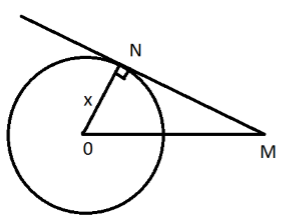×#### Thank you for registering.

One of our academic counsellors will contact you within 1 working day.

Click to Chat

1800-1023-196

+91-120-4616500

CART 0

• 0

MY CART (5)

Use Coupon: CART20 and get 20% off on all online Study Material

ITEM
DETAILS
MRP
DISCOUNT
FINAL PRICE
Total Price: Rs.

There are no items in this cart.
Continue Shopping• Complete JEE Main/Advanced Course and Test Series
• OFFERED PRICE: Rs. 15,900
• View Details

```Chapter 10: Circles Exercise – 10.1

Question: 1

Fill in the blanks:

(i) The common point of tangent and the circle is called _________.

(ii) A circle may have _____ parallel tangent.

(iii) A tangent to a circle intersects it in ______ point.

(iv) A line intersecting a circle in two points is called a _______

(v) The angle between tangent at a point P on circle and radius through the point is _______

Solution:

(i) point of contact.

(ii) two

(iii) one

(iv) secant.

(v) 90°.

Question: 2

How many tangents can a circle have?

Solution:

Tangent: Aline intersecting circle in one point is called a tangent As there are infinite number of points on the circle, a circle has many (infinite) tangents.

Question: 3

O is the centre the circle shown below with a radius of 8 cm. The circle cuts the tangent AB through O at B such that AB = 15 cm. Find OB.Solution:

Given data: AB = 15 cm OA = 8 cm (radius of the circle) we know that: the tangent cuts the circle at 90 degrees. Therefore, OA is the hypotenuse of the triangle OAB. Hence, the longest side can be found by using Pythagoras Theorem. We have, OB = 17 cm Therefore, OB = 17 cm

Question: 4

If the tangent at point P to the circle with centre O cuts a line through O at Q such that PQ = 24 cm and OQ = 25 cm. Find the radius of the circle.

Solution:

Given data: PQ = 24 cm OQ = 25 cm OP = radius = ?P is a point of contact, at point of contact, tangent and radius are perpendicular to each other. Therefore triangle is right angled triangle angle OPQ = 90° BY Pythagoras theorem, OP = 7 cm Therefore, OP = radius = 7 cm
```### Course Features

• 728 Video Lectures
• Revision Notes
• Previous Year Papers
• Mind Map
• Study Planner
• NCERT Solutions
• Discussion Forum
• Test paper with Video Solution